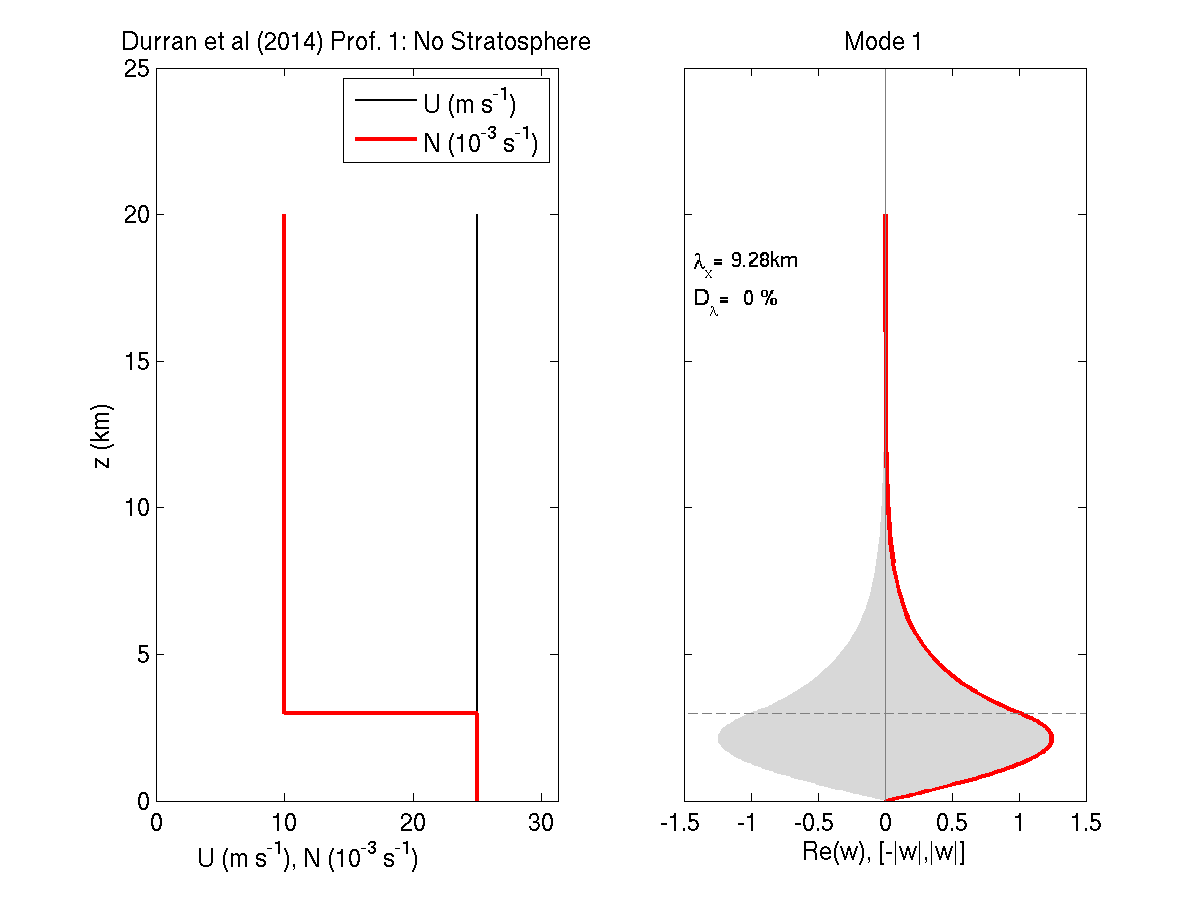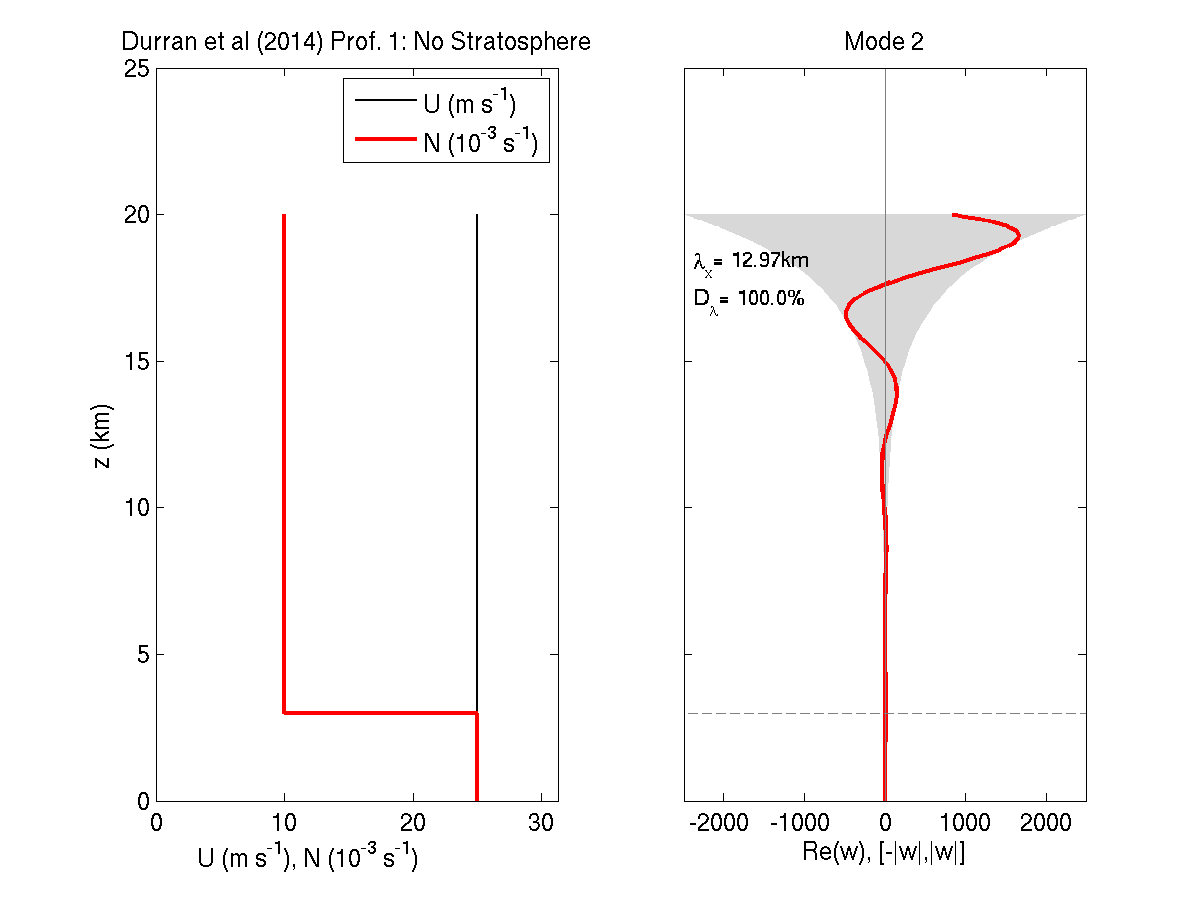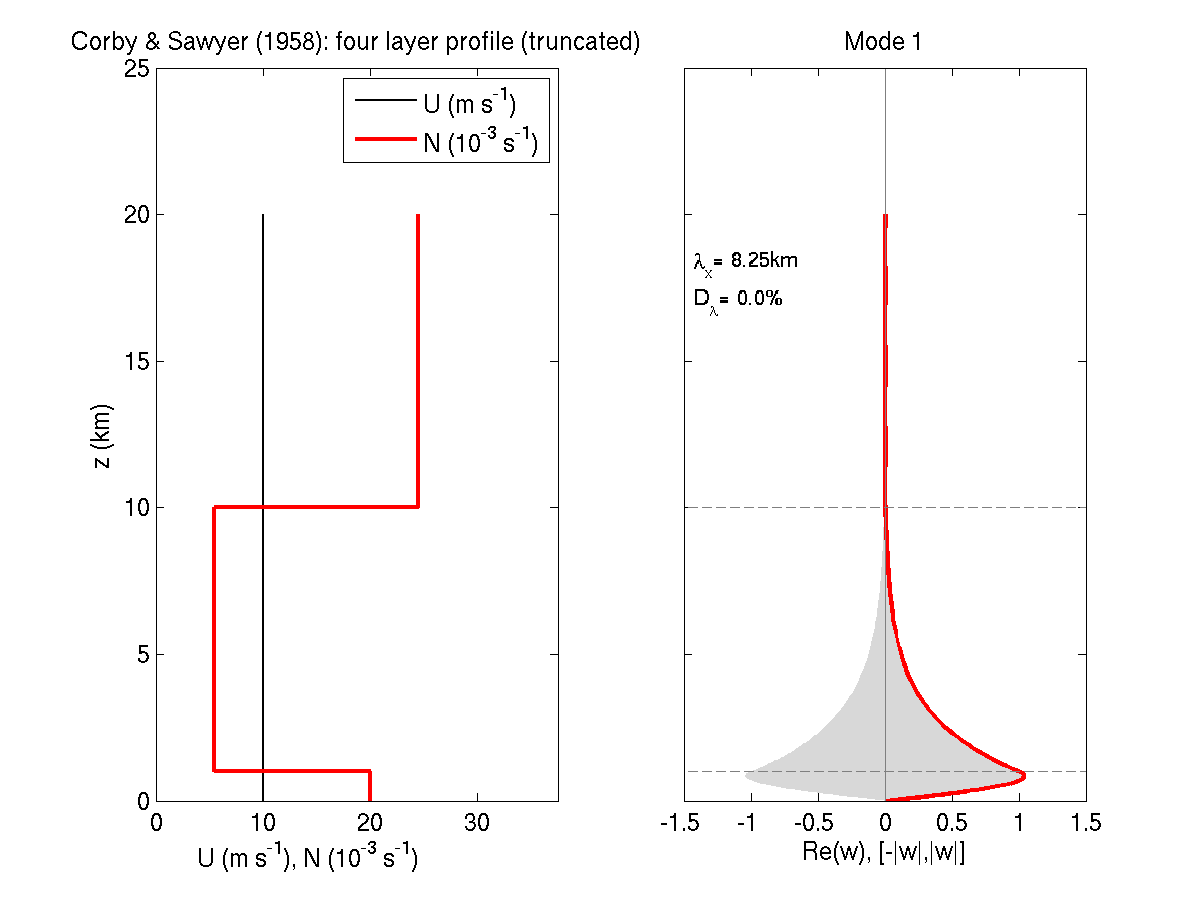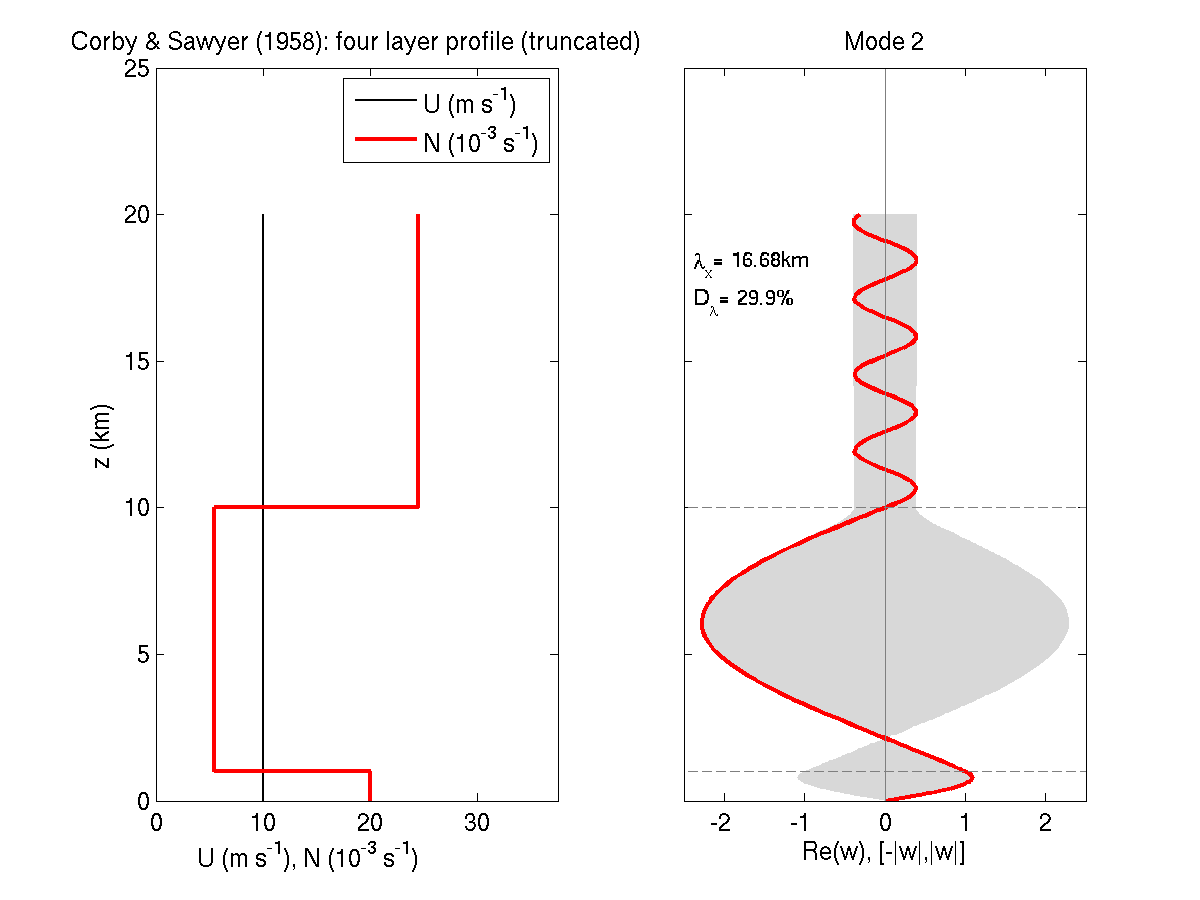# MATLAB Codes for computing trapped mountain waves

MATLAB codes that compute linear modes that represent trapped waves in the lee of mountain barriers can be found here.
These routines search for solutions to the nonlinear eigenvalue problem described in section 2 of Durran et al (2014) using the numerical method in the appendix of that paper. Any questions or comments should be directed to Peter Blossey (pblossey@uw.edu).

### An example from Durran et al (2014)

By running the script TrappedWavesExample in MATLAB, the method can be demonstrated on a number of the basic state profiles in Durran et al (2014). For example, running with icase = 1 yields:

``` >> TrappedWaveExample ====================================================== Searching for trapped or leaky trapped wave modes for: Durran et al (2014) Prof. 1: No Stratosphere ... Note that we are searching a wide variety of parameter space for solutions so that error messages may appear below ... ... ```
Many error messages are produced because we are trying many potential solutions for the nonlinear eigenvalue problem and not all of them are appropriate.
The few (if any) that satisfy the eigenvalue problem are returned below.

``` Found 2 solutions for Durran et al (2014) Prof. 1: No Stratosphere Mode 1: eigenvalue = 6.7671e-04 + 1i* 0.0000e+00, lambda x = 9.28 km, convergence residual = 1.2584e-12 Mode 2: eigenvalue = 4.8429e-04 + 1i* 1.1216e-03, lambda x = 12.97 km, convergence residual = 9.3655e-11 ```

Here, two solutions have been found. The first one, a purely trapped lee wave (Mode 1), has a wavelength of 9.28km and is familiar from figure 2 of Durran et al (2014). The second mode is a leaky trapped wave that decays strongly downstream (losing all of its amplitude within one wavelength). The vertical structure of base state and the two trapped wave solutions are shown in figures:Because the second mode (at right) decays so strongly downstream, the linear trapped wave response would be dominated by Mode 1 if that mode is excited by the flow over a mountain.

The MATLAB script `TrappedWaveExample.m` supplies ten ready-made base states and find trapped wave solutions for each, sometimes only one mode, and other times more than one. Try them all by changing the limits on the `for icase =` loop at the top of the script.

### Revisiting Corby & Sawyer (1958)

Even better, look for trapped wave solutions for a different basic state. You just need to specify a profile that is piecewise linear in U, piecewise constant in Brunt-Vaisala frequency N and has uniform velocity over a layer at the top of the model. The last restriction makes possible the application of our wave radiation condition at the top of the profile.

As an example, let's revisit Corby and Sawyer (1958) and see if we can approximately reproduce the wavelenths of the three modes in their figure 4. Let's build a three-layer approximation to their four layer atmosphere -- our radiation condition should let us cut off the profile in the third layer. Let's use the following heights with vertically-uniform wind and the Brunt-Vaisala frequencies (N^2 = l^2 * U^2, where l is the Scorer parameter):

``` H = [0 1e3 10e3 20e3]; Ubar = [10 10 10 10]; Nbrunt = sqrt(100*[4e-6 0.3e-6 6e-6]);```

Note that we only need to specify three values of N because it is uniform betwee the four heights specified in H. Also, note that Corby and Sawyer only specified values for the Scorer parameter, so that we can freely choose the (vertically-uniform) wind speed as long as l = N/U. This base state is available under `icase=11` in `TrappedWaveExample.m`. Trying this yields:

``` Found 2 solutions for Corby & Sawyer (1958): four layer profile (truncated) Mode 1: eigenvalue = 7.6142e-04 + 1i* 1.4088e-08, lambda x = 8.25 km, convergence residual = 8.4203e-13 Mode 2: eigenvalue = 3.7669e-04 + 1i* 2.1257e-05, lambda x = 16.68 km, convergence residual = 2.3472e-12 ```

so that we have found two modes. The first corresponds almost exactly with the first mode in Corby and Sawyer (1958), matching all four given digits of the wavenumber k (which they specify as k=0.7614 km^(-1)). The form of the second mode is very similar to that of their third mode, but our wavenumber is slightly larger, perhaps because we have truncated the base state at 20km height in the stratosphere and applied a radiation condition there. The two modes, shown below may be compared with figure 4a in Corby and Sawyer.Note that our method only predicts the form, wavelength and downstream decay rate of the trapped wave modes. A different method would be required to predict their amplitude.